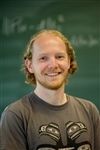# Tristan van Leeuwen (UU)Date: 12 October 2016

Time: 12:45 - 13:30 (Lunch available from 12:35)

Room: RA 1501 (Ravelijn)

## Title: Joint parameter and state estimation for inverse problemsics

Abstract:

In many applications, such as exploration geophysics, seismology and ultrasound imaging, we want to estimate material properties from indirect observations. We can pose the inverse problem as a non-linear data-fitting problem: fit the coefficients of a partial differential equation (PDE) such that its solution fits the observations approximately. This allows one to effectively deal with errors in the observations.
However, the PDE most likely does not represent the physics properly. For example, the equation may only capture part of the physics or the source-term may include (unknown) random effects. In such cases, it is not desirable to solve the PDE exactly. Instead, we can formulate a joint estimation problem: find the field and the coefficients such that they obey both physics and observations approximately. In this formulation we put the physics and the observations on equal footing, allowing both errors in the model as well as the observations.
In this talk, I discuss the implications of such a joint approach as well as practical algorithms and ways to learn the covariance matrices corresponding to errors in the observations and the physics.